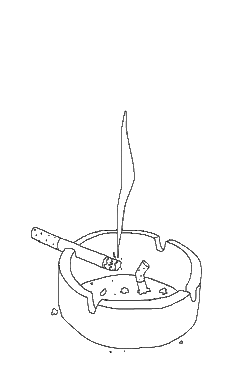# August 2020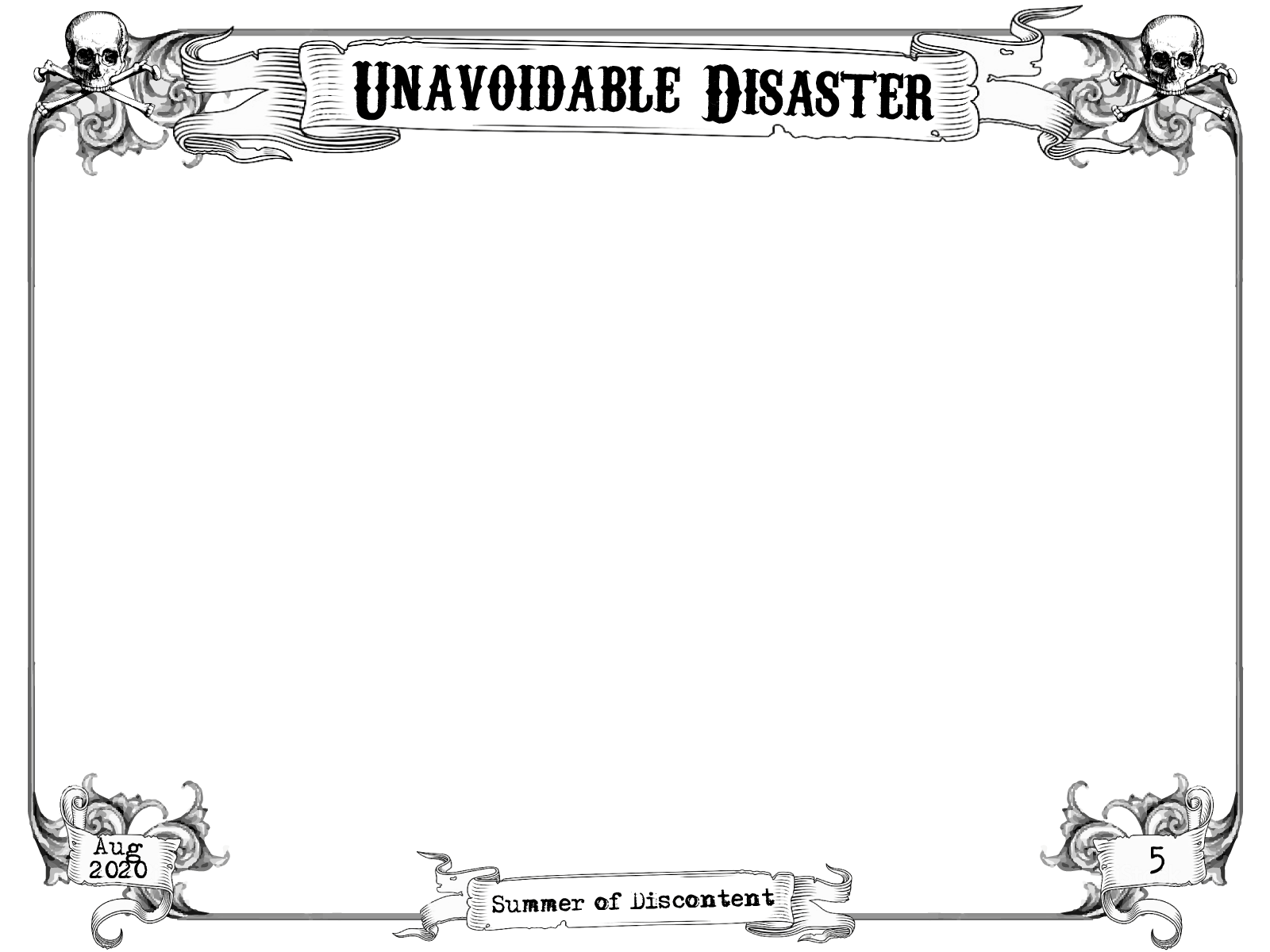xxxxx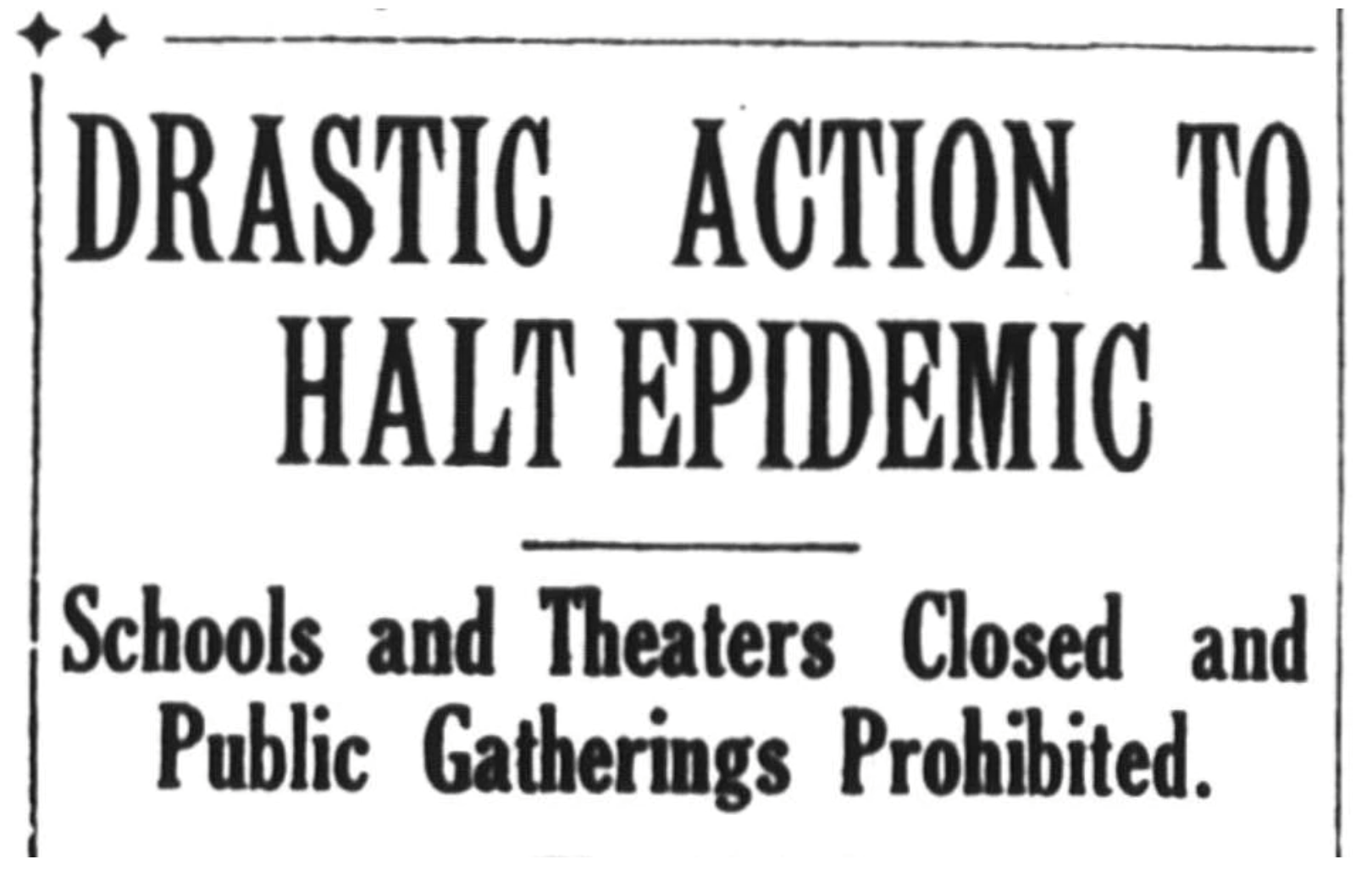x
Page 2xxxxxx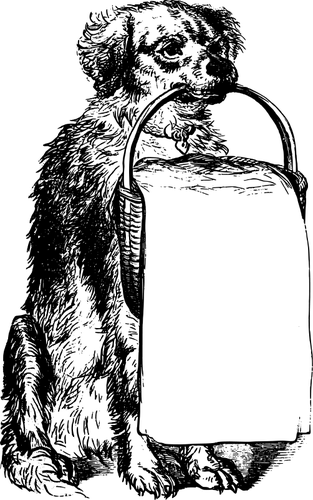Page 3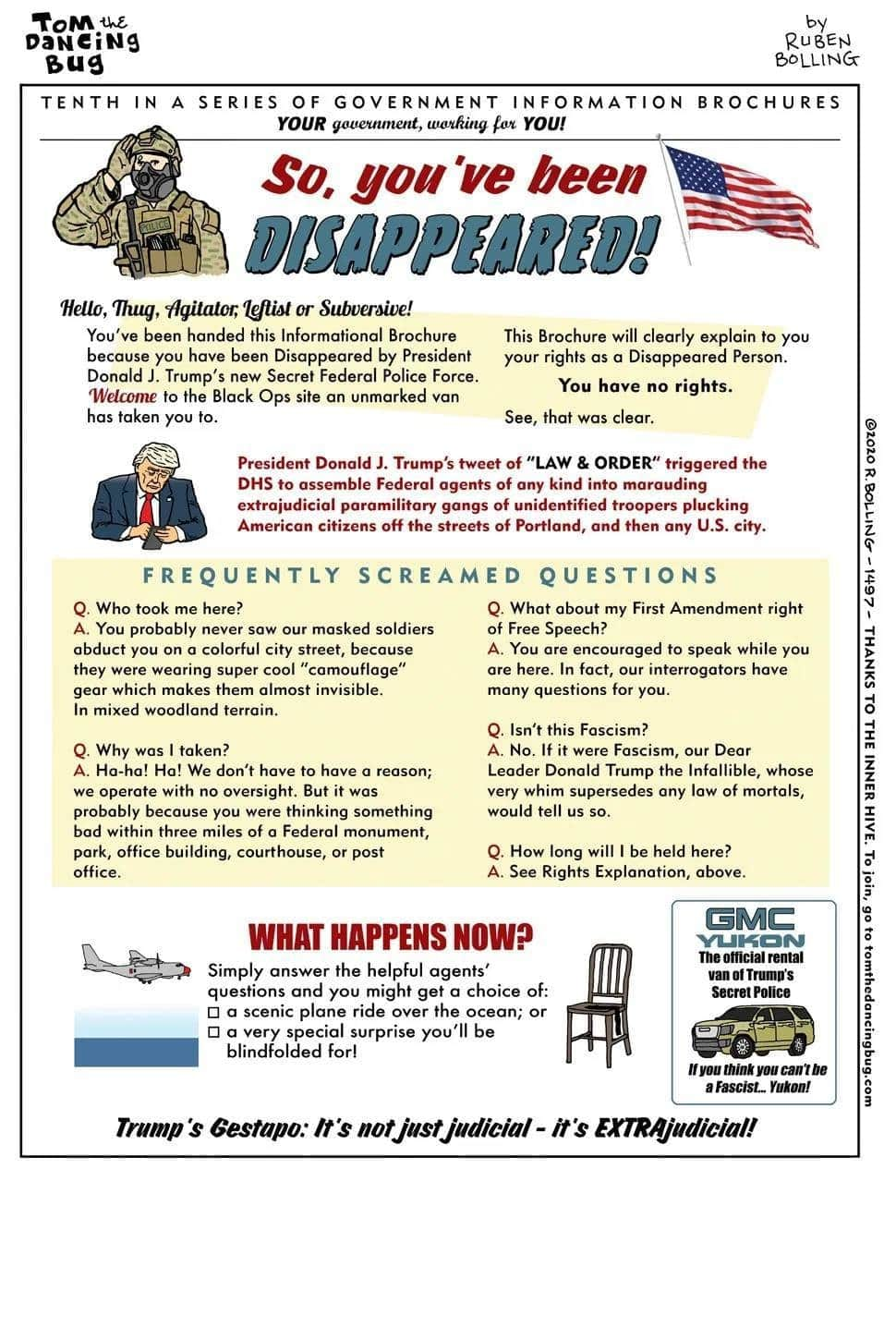xxxxx
Page 4xxxxxPage 5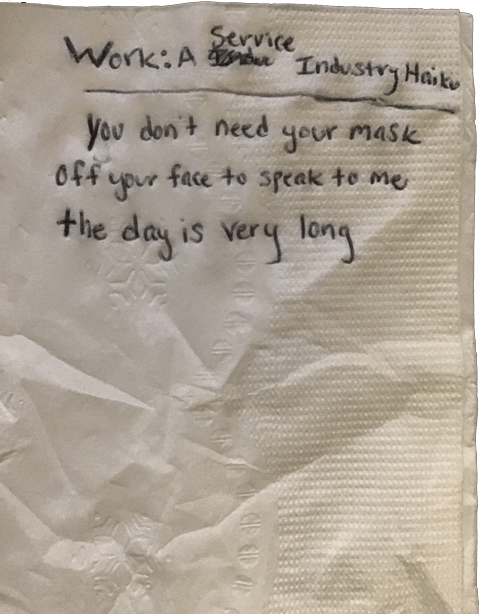xxxxxxx
Page 6xxxxxxx
Page 7xxx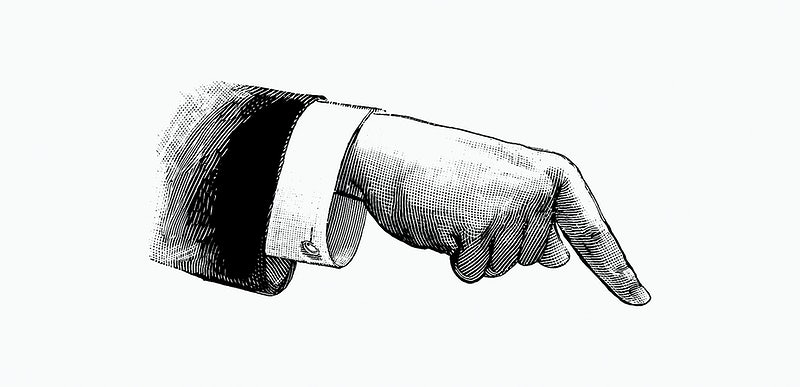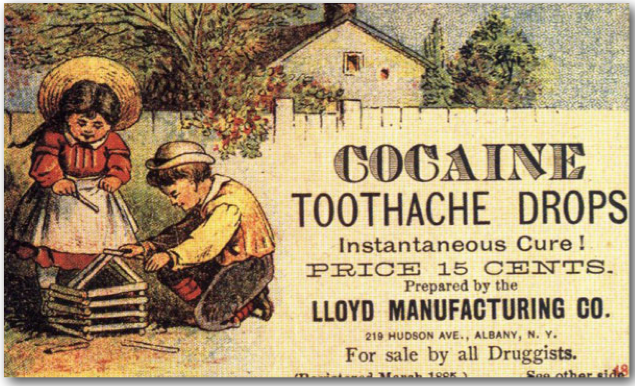xxx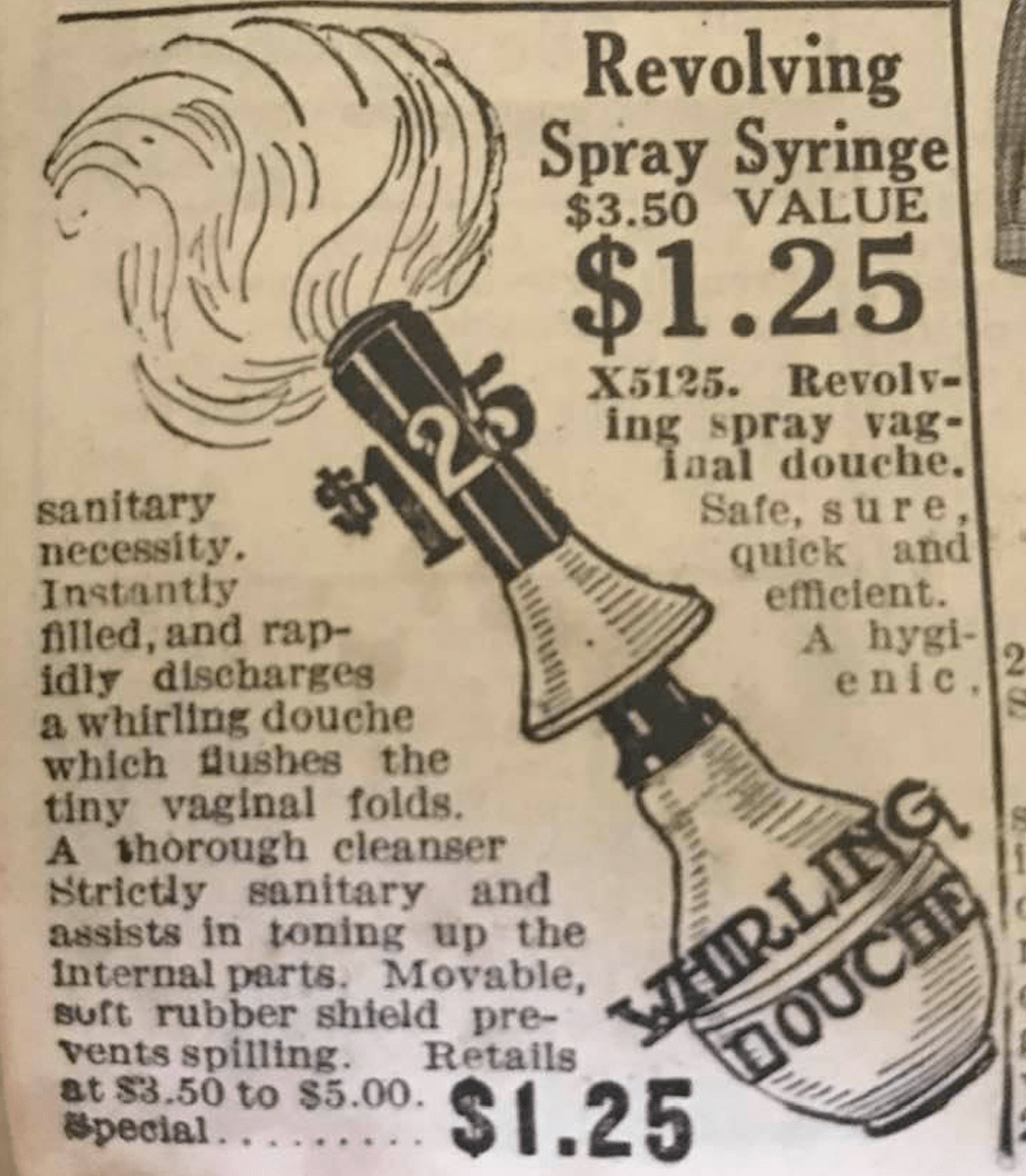xPage 8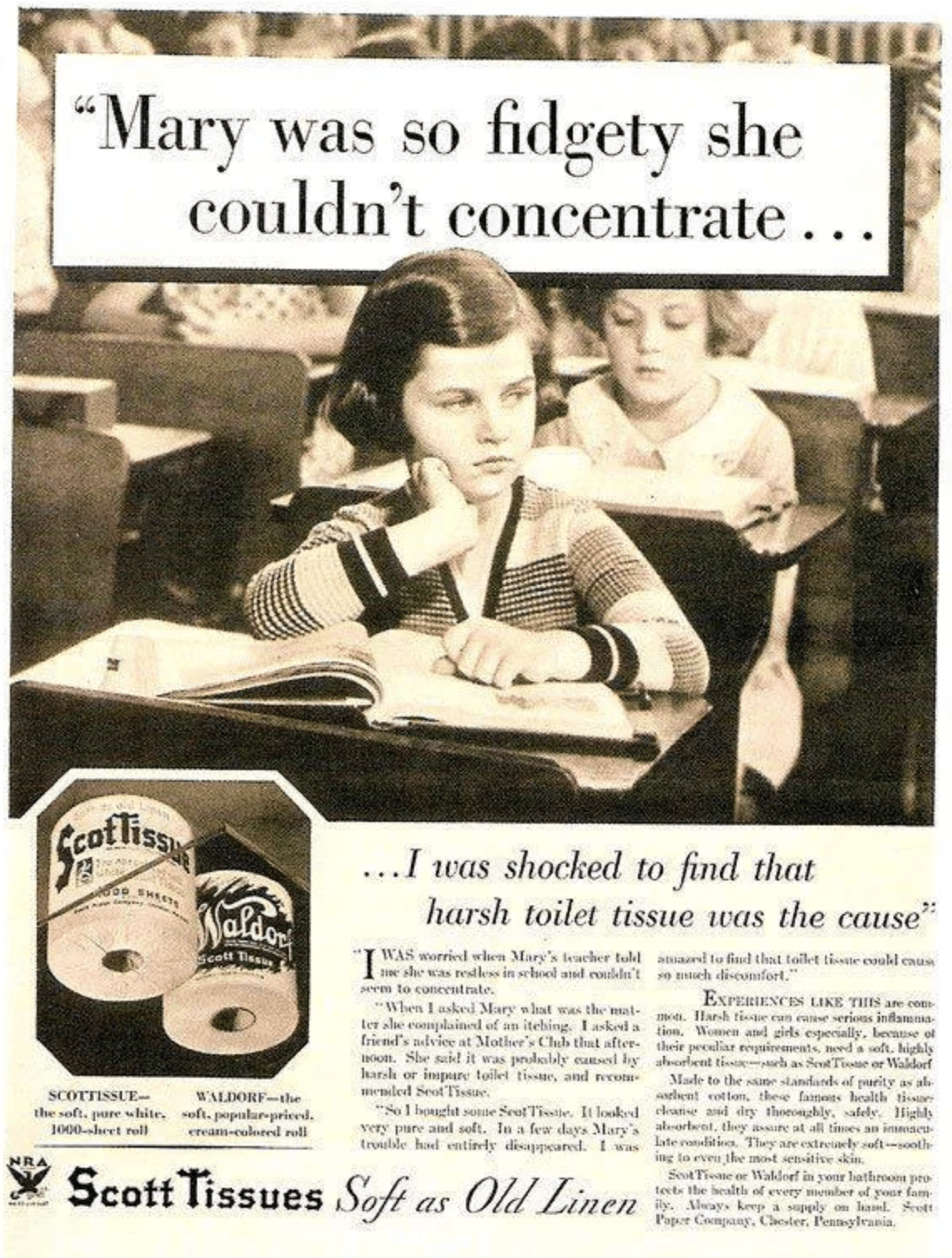xx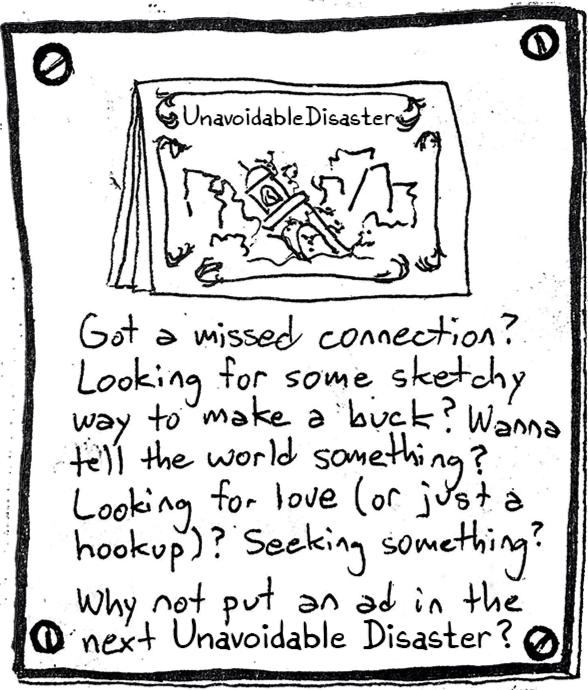xxxxPage 9
8+